# Common Core: High School - Geometry : Congruence

## Example Questions

### Example Question #131 : Congruence

How is a square altered to result in a rhombus?

Change the length of the sides

A square can never be a rhombus

Change the vertical distance between the two horizontal lines.

Rotate the squareChange the interior angles.

Change the interior angles.

Explanation:

Both squares and rhombi are quadrilaterals and parallelograms. Quadrilaterals are four sided figures and parallelograms are figures that have opposite sides that are parallel.

Squares by definition contain fourangles; rhombi on the other hand have two sets of opposite congruent angles. Therefore, for a square to to altered into a rhombus, the interior angles must be altered.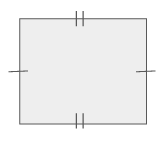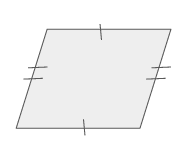### Example Question #131 : Congruence

Given two rays, how can a line be constructed?

Two rays cannot be constructed into one line.

Connect the vertices of the rays and have their directions pointfrom each other.

Connect the vertices of the rays and have their directions pointfrom each other.

Have their directions pointfrom each other.

None of the other answers.

Connect the vertices of the rays and have their directions pointfrom each other.

Explanation:

Recall that a ray is a line segment that has a vertex at one end and extends at the other. The extension is depicted by an arrow. In order for two rays to become one line segment the vertices must be connected and the arrows going in the opposite direction. For the rays to be going in the opposite direction they must haveangle between them.

Therefore, the correct answer is

"Connect the vertices of the rays and have their directions pointfrom each other."

### Example Question #1 : Construct Inscribed Figures (Equilateral Triangles, Squares, Regular Hexagons): Ccss.Math.Content.Hsg Co.D.13

Given only the line segment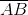, what is the first step to construct a diamond.

Draw a perpendicular line from pointof length.

Calculate the slope of.

Calculate the length of.

Draw aangle.

Draw a parallel line from pointof length.

Calculate the length of.

Explanation:

Given only a line segment there are numerous steps that need to be taken to construct a diamond. Recall a diamond is a parallelogram with four equilateral sides where the diagonals are vertical and horizontal.

The first step in constructing a diamond would be to calculate the length of the line segment. After the length is known then the slope can be found and from there, each of the connecting lines can be drawn.

Therefore, the correct answer is,

"Calculate the length of."

### Example Question #131 : High School: Geometry

Which of the following images is a triangle that is inscribed in a circle?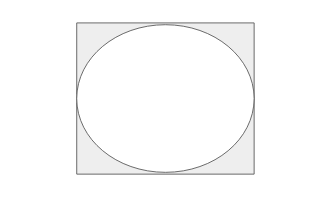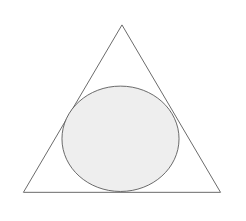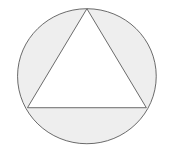None of the answers.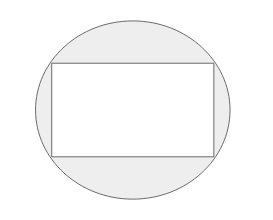Explanation:

To determine which image illustrates a triangle that is inscribed in a circle, first understand what the term "inscribed" means.

"Inscribed" means to draw inside of. Therefore, a triangle inscribed in a circle means the triangle will be drawn inside of a circle.

The image that illustrates this is as followed.### Example Question #1 : Construct Inscribed Figures (Equilateral Triangles, Squares, Regular Hexagons): Ccss.Math.Content.Hsg Co.D.13

Which of the following images is a rectangle that is inscribed in a circle?None of the other answers.Explanation:

To determine which image illustrates a rectangle that is inscribed in a circle, first understand what the term "inscribed" means.

"Inscribed" means to draw inside of. Therefore, a rectangle inscribed in a circle means the rectangle will be drawn inside of a circle.

The image that illustrates this is as followed.### Example Question #131 : Congruence

Which of the following images is a circle that is inscribed in a rectangle?None of the other answers.Explanation:

To determine which image illustrates a circle that is inscribed in a rectangle, first understand what the term "inscribed" means.

"Inscribed" means to draw inside of. Therefore, a circle inscribed in a rectangle means the circle will be drawn inside of a rectangle.

The image that illustrates this is as followed.### Example Question #5 : Construct Inscribed Figures (Equilateral Triangles, Squares, Regular Hexagons): Ccss.Math.Content.Hsg Co.D.13

Which of the following images is a circle that is inscribed in a triangle?None of the answers.Explanation:

To determine which image illustrates a circle that is inscribed in a triangle, first understand what the term "inscribed" means.

"Inscribed" means to draw inside of. Therefore, a circle inscribed in a triangle means the circle will be drawn inside of a triangle.

The image that illustrates this is as followed.### Example Question #6 : Construct Inscribed Figures (Equilateral Triangles, Squares, Regular Hexagons): Ccss.Math.Content.Hsg Co.D.13

Which of the following images is a pentagon that is inscribed in a circle?Explanation:

To determine which image illustrates a pentagon that is inscribed in a circle, first understand what the term "inscribed" means.

"Inscribed" means to draw inside of. Therefore, a pentagon inscribed in a circle means the pentagon will be drawn inside of a circle.

The image that illustrates this is as followed.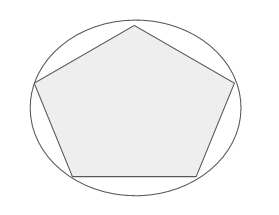### Example Question #7 : Construct Inscribed Figures (Equilateral Triangles, Squares, Regular Hexagons): Ccss.Math.Content.Hsg Co.D.13

Which of the following images is a circle that is inscribed in a pentagon?Explanation:

To determine which image illustrates a circle that is inscribed in a pentagon, first understand what the term "inscribed" means.

"Inscribed" means to draw inside of. Therefore, a circle inscribed in a pentagon means the circle will be drawn inside of a pentagon.

The image that illustrates this is as followed.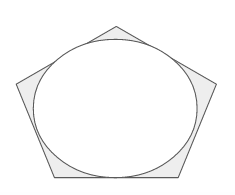### Example Question #8 : Construct Inscribed Figures (Equilateral Triangles, Squares, Regular Hexagons): Ccss.Math.Content.Hsg Co.D.13

If a rectangle is inscribed in a circle what can be said about the relationship between the circles diameter and a measurement on the rectangle?

The diameter of the circle is equal to the side squared of the rectangle.

All of the answers are equivalencies.

The diameter of the circle is equal to the side length of the rectangle.

The diameter of the circle is equal to the perimeter of the rectangle.

The diameter of the circle is equal to the diagonal of the rectangle.

The diameter of the circle is equal to the diagonal of the rectangle.

Explanation:

First understand what the term "inscribed" means.

"Inscribed" means to draw inside of. Therefore, a rectangle inscribed in a circle means the rectangle will be drawn inside of a circle.

From here the following image can be constructed.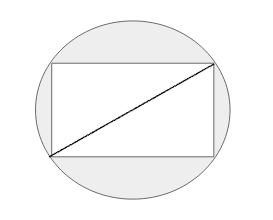The diameter of the circle is any and all straight lines that cut the circle in half. Since the rectangle is inscribed in that circle and all corners touch the circle then the diameter of the circle is equivalent to the length of the rectangle's diagonal.

### All Common Core: High School - Geometry Resources